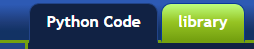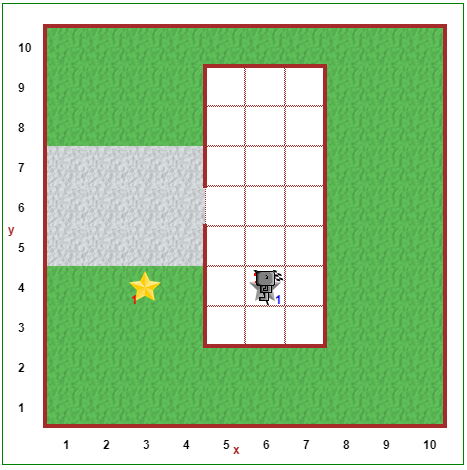# Step 6: Saving Functions in the Library¶

## Tutorial¶

In addition to having Reeborg making right turns, you might have had it turn around a few times; by turning around, I mean changing direction so that Reeborg is heading back to where it was coming from. We can define a function `turn_around()` as follows:

```def turn_around():
turn_left()
turn_left()
```

When programmer make use of a given function in different programs, rather than redefining it in each program they write, they put them in special programs called libraries and they have a way to ensure that their other programs can use the functions that are found in the library.

You are going to use the function `turn_right()` a lot! Instead of rewriting it each time, what you are going to do is to write it once (more) but, this time, instead of writing it in the editor with the Python Code tab, you will click on the library tab and write it there. Oh, and you should also define `turn_around()` there as well.Then, when you want to use the functions defined in your library, you will simply type `from library import` (followed by the function names, separated by commas) on its own line in the Python Code editor. For example, if you have defined `turn_right()` in the library, you could type `from library import turn_right`, and you would now be able to use the `turn_right()` function anywhere in your code. See the example below:

```# import functions from the library tab
from library import turn_right, turn_around

# when you want to use the functions
turn_right()
turn_around()
```

If you end up saving a large number of functions into the library, you may also import all of the functions in the library by calling `import library`. When you do this, however, be aware that you then need to specify where the function is coming from when you call it. For example, if we had defined `turn_right()` in the library, we would call the function using `library.turn_right()`. See the example below:

```# import functions from the library tab
import library

# when you want to use the function
library.turn_right()
library.turn_around()
```

If you want to, you can save yourself a few keystrokes by renaming the library tab when you import it. Consider the following example:

```# import functions from the library tab
import library as lib

# when you want to use the function
lib.turn_right()
lib.turn_around()
```

Caution

Although you may see it in some tutorials, you should avoid using wildcard import statements like `from library import *`. Although this imports all the functions in the library, it can cause problems with your code, since what you import may clash with existing code. This is sometimes called namespace pollution.Reeborg needs to go pick up the newspaper (The Star Phoenix) that is sitting outside his house. If you haven’t done it yet, define both the `turn_right()` and `turn_around()` functions in the library tab. Now use the functions defined in the library to have Reeborg pick up the newspaper, walk back into the house, and put the newspaper down. Once again, be sure to use comments and whitespace to increase the readability of your solution!